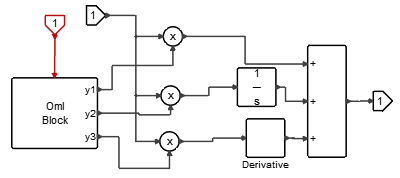# RunTimePID

This block implements a tunable PID. During the simulation, the coefficients of the PID can be manually tuned.
The current value of the gain is equal to the Value multiplied by the Factor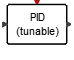Dynamical

## Description

The RunTimePID block implements a PID controller. The PID controller calculation (algorithm) involves three separate parameters: Proportional, Integral and Derivative. The Proportional value determines the reaction to the current error, the Integral value determines the reaction based on the sum of recent errors, and the Derivative value determines the reaction to the rate at which the error has been changing. The weighted sum of these three actions is used to adjust the process via a control element such as the position of a control valve or the power supply of a heating element. The value of the parameters can be tuned during the simulation.

The left end of slider represents the Min value.
The right end of slider represents the Max value.
The number of ticks is (Max-Min)/Step.
The slider corresponds to a nominal value based on its position with regard to ticks.
The real PID parameter is calculated by Value*Factor.

## Parameters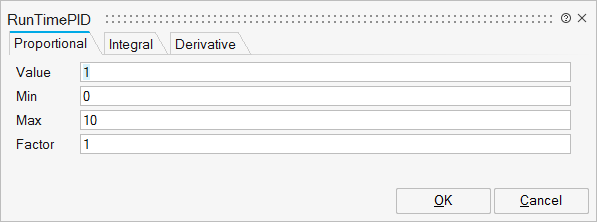NameLabelDescriptionData TypeValid Values

p_value

Value

Int value. The current value of the slider.

Scalar

p_min

Min

Int value. The minimum value of the slider.

Scalar

p_max

Max

Int value. The maximum value of the slider.

Scalar

p_step

Step

Int value. The step taken by the slider.

Scalar

p_factor

Factor

Real Value. the factor used to convert the slider value to the gain value.

Scalar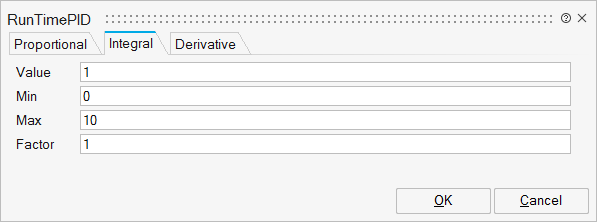NameLabelDescriptionData TypeValid Values

i_value

Value

Int value. The current value of the slider.

Scalar

i_min

Min

Int value. The minimum value of the slider.

Scalar

i_max

Max

Int value. The maximum value of the slider.

Scalar

i_step

Step

Int value. The step taken by the slider.

Scalar

i_factor

Factor

Real Value. the factor used to convert the slider value to the gain value.

Scalar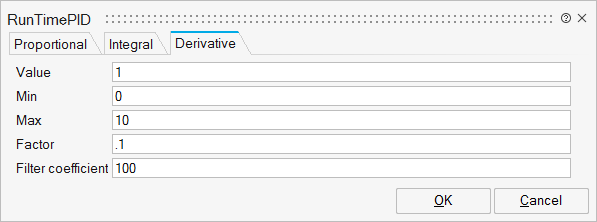NameLabelDescriptionData TypeValid Values

d_value

Value

Int value. The current value of the slider.

Scalar

d_min

Min

Int value. The minimum value of the slider.

Scalar

d_max

Max

Int value. The maximum value of the slider.

Scalar

d_step

Step

Int value. The step taken by the slider.

Scalar

d_factor

Factor

Real Value. the factor used to convert the slider value to the gain value.

Scalar

N

Filter coefficient

Scalar

## Ports

NameTypeDescriptionIO TypeNumber

Port 1

explicit

input

1

Port 2

explicit

output

1

Port 3

activation

input

1

NameValueDescription

always active

yes

direct-feedthrough

yes

PID block is direct-feedthrough unless the proportional gain (Kp) is zero (0).

zero-crossing

no

mode

no

continuous-time state

no

discrete-time state

no

## Diagram